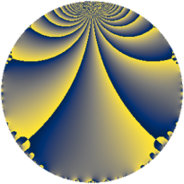# Properties

 Label 7728.2.a.bbLevel $7728$ Weight $2$ Character orbit 7728.a Self dual yes Analytic conductor $61.708$ Analytic rank $1$ Dimension $2$ CM no Inner twists $1$

# Related objects

## Newspace parameters

 Level: $$N$$ $$=$$ $$7728 = 2^{4} \cdot 3 \cdot 7 \cdot 23$$ Weight: $$k$$ $$=$$ $$2$$ Character orbit: $$[\chi]$$ $$=$$ 7728.a (trivial)

## Newform invariants

 Self dual: yes Analytic conductor: $$61.7083906820$$ Analytic rank: $$1$$ Dimension: $$2$$ Coefficient field: $$\Q(\sqrt{5})$$ Defining polynomial: $$x^{2} - x - 1$$ Coefficient ring: $$\Z[a_1, \ldots, a_{13}]$$ Coefficient ring index: $$1$$ Twist minimal: no (minimal twist has level 1932) Fricke sign: $$1$$ Sato-Tate group: $\mathrm{SU}(2)$

## $q$-expansion

Coefficients of the $$q$$-expansion are expressed in terms of $$\beta = \frac{1}{2}(1 + \sqrt{5})$$. We also show the integral $$q$$-expansion of the trace form.

 $$f(q)$$ $$=$$ $$q - q^{3} + ( -1 + 3 \beta ) q^{5} - q^{7} + q^{9} +O(q^{10})$$ $$q - q^{3} + ( -1 + 3 \beta ) q^{5} - q^{7} + q^{9} -3 q^{11} + ( -2 + \beta ) q^{13} + ( 1 - 3 \beta ) q^{15} + ( 5 - 4 \beta ) q^{17} + ( 3 - 2 \beta ) q^{19} + q^{21} + q^{23} + ( 5 + 3 \beta ) q^{25} - q^{27} -5 q^{29} + ( -9 + 2 \beta ) q^{31} + 3 q^{33} + ( 1 - 3 \beta ) q^{35} + ( -1 - 2 \beta ) q^{37} + ( 2 - \beta ) q^{39} + 3 q^{41} + \beta q^{43} + ( -1 + 3 \beta ) q^{45} + 2 \beta q^{47} + q^{49} + ( -5 + 4 \beta ) q^{51} + ( 9 - 7 \beta ) q^{53} + ( 3 - 9 \beta ) q^{55} + ( -3 + 2 \beta ) q^{57} + ( -1 + 9 \beta ) q^{59} -3 \beta q^{61} - q^{63} + ( 5 - 4 \beta ) q^{65} + ( 9 - 7 \beta ) q^{67} - q^{69} + ( -6 - \beta ) q^{71} + ( 7 - 8 \beta ) q^{73} + ( -5 - 3 \beta ) q^{75} + 3 q^{77} + ( 3 + 4 \beta ) q^{79} + q^{81} + ( -9 - 2 \beta ) q^{83} + ( -17 + 7 \beta ) q^{85} + 5 q^{87} + ( 8 - 9 \beta ) q^{89} + ( 2 - \beta ) q^{91} + ( 9 - 2 \beta ) q^{93} + ( -9 + 5 \beta ) q^{95} + ( 11 - 8 \beta ) q^{97} -3 q^{99} +O(q^{100})$$ $$\operatorname{Tr}(f)(q)$$ $$=$$ $$2q - 2q^{3} + q^{5} - 2q^{7} + 2q^{9} + O(q^{10})$$ $$2q - 2q^{3} + q^{5} - 2q^{7} + 2q^{9} - 6q^{11} - 3q^{13} - q^{15} + 6q^{17} + 4q^{19} + 2q^{21} + 2q^{23} + 13q^{25} - 2q^{27} - 10q^{29} - 16q^{31} + 6q^{33} - q^{35} - 4q^{37} + 3q^{39} + 6q^{41} + q^{43} + q^{45} + 2q^{47} + 2q^{49} - 6q^{51} + 11q^{53} - 3q^{55} - 4q^{57} + 7q^{59} - 3q^{61} - 2q^{63} + 6q^{65} + 11q^{67} - 2q^{69} - 13q^{71} + 6q^{73} - 13q^{75} + 6q^{77} + 10q^{79} + 2q^{81} - 20q^{83} - 27q^{85} + 10q^{87} + 7q^{89} + 3q^{91} + 16q^{93} - 13q^{95} + 14q^{97} - 6q^{99} + O(q^{100})$$

## Embeddings

For each embedding $$\iota_m$$ of the coefficient field, the values $$\iota_m(a_n)$$ are shown below.

For more information on an embedded modular form you can click on its label.

Label $$\iota_m(\nu)$$ $$a_{2}$$ $$a_{3}$$ $$a_{4}$$ $$a_{5}$$ $$a_{6}$$ $$a_{7}$$ $$a_{8}$$ $$a_{9}$$ $$a_{10}$$
1.1
 −0.618034 1.61803
0 −1.00000 0 −2.85410 0 −1.00000 0 1.00000 0
1.2 0 −1.00000 0 3.85410 0 −1.00000 0 1.00000 0
 $$n$$: e.g. 2-40 or 990-1000 Significant digits: Format: Complex embeddings Normalized embeddings Satake parameters Satake angles

## Atkin-Lehner signs

$$p$$ Sign
$$2$$ $$-1$$
$$3$$ $$1$$
$$7$$ $$1$$
$$23$$ $$-1$$

## Inner twists

This newform does not admit any (nontrivial) inner twists.

## Twists

By twisting character orbit
Char Parity Ord Mult Type Twist Min Dim
1.a even 1 1 trivial 7728.2.a.bb 2
4.b odd 2 1 1932.2.a.h 2
12.b even 2 1 5796.2.a.j 2

By twisted newform orbit
Twist Min Dim Char Parity Ord Mult Type
1932.2.a.h 2 4.b odd 2 1
5796.2.a.j 2 12.b even 2 1
7728.2.a.bb 2 1.a even 1 1 trivial

## Hecke kernels

This newform subspace can be constructed as the intersection of the kernels of the following linear operators acting on $$S_{2}^{\mathrm{new}}(\Gamma_0(7728))$$:

 $$T_{5}^{2} - T_{5} - 11$$ $$T_{11} + 3$$ $$T_{13}^{2} + 3 T_{13} + 1$$ $$T_{17}^{2} - 6 T_{17} - 11$$

## Hecke characteristic polynomials

$p$ $F_p(T)$
$2$ $$T^{2}$$
$3$ $$( 1 + T )^{2}$$
$5$ $$-11 - T + T^{2}$$
$7$ $$( 1 + T )^{2}$$
$11$ $$( 3 + T )^{2}$$
$13$ $$1 + 3 T + T^{2}$$
$17$ $$-11 - 6 T + T^{2}$$
$19$ $$-1 - 4 T + T^{2}$$
$23$ $$( -1 + T )^{2}$$
$29$ $$( 5 + T )^{2}$$
$31$ $$59 + 16 T + T^{2}$$
$37$ $$-1 + 4 T + T^{2}$$
$41$ $$( -3 + T )^{2}$$
$43$ $$-1 - T + T^{2}$$
$47$ $$-4 - 2 T + T^{2}$$
$53$ $$-31 - 11 T + T^{2}$$
$59$ $$-89 - 7 T + T^{2}$$
$61$ $$-9 + 3 T + T^{2}$$
$67$ $$-31 - 11 T + T^{2}$$
$71$ $$41 + 13 T + T^{2}$$
$73$ $$-71 - 6 T + T^{2}$$
$79$ $$5 - 10 T + T^{2}$$
$83$ $$95 + 20 T + T^{2}$$
$89$ $$-89 - 7 T + T^{2}$$
$97$ $$-31 - 14 T + T^{2}$$